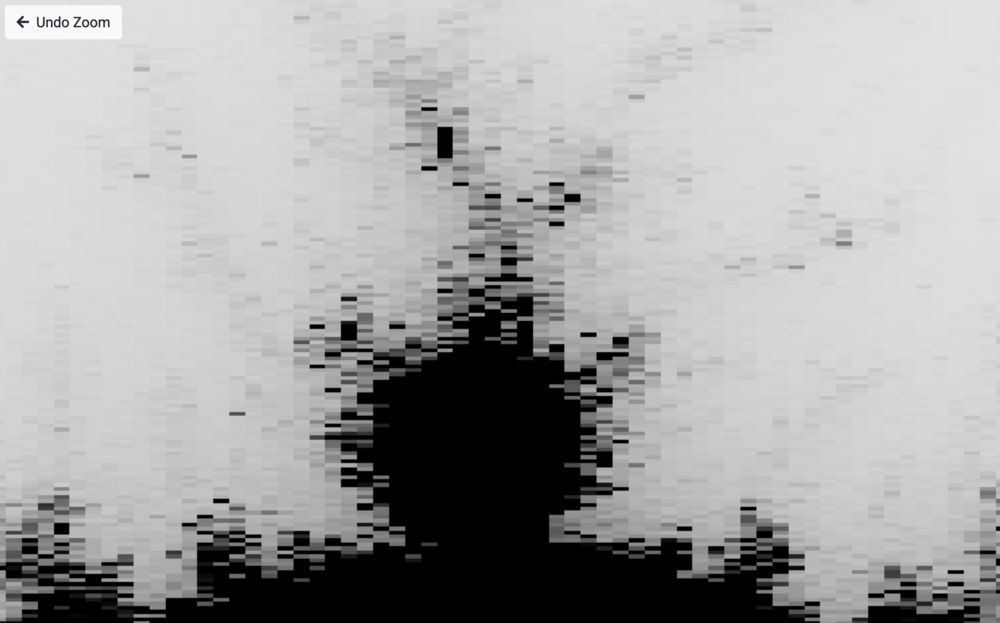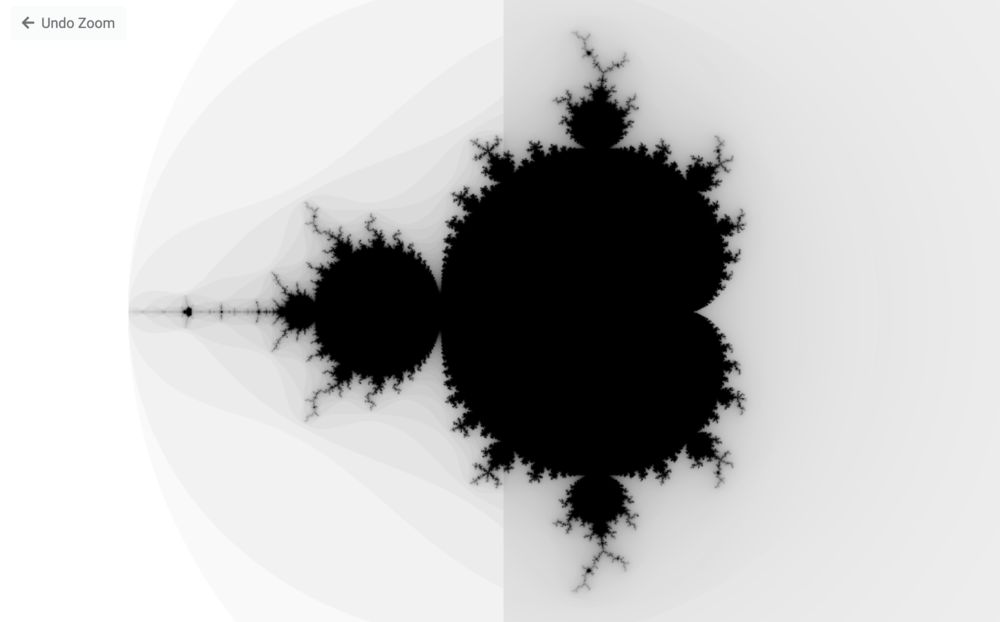## What is a fractal?

The first thing you might ask is "what is a fractal?"

You can have a look at this little project I created to get an idea.

"Fractal" is a pretty general term which covers a lot of different self-similar shapes. Self-similar meaning that they look similar at different magnification levels.

They also tend to include shapes which look pretty crazy, but are based on relatively simple iterative mathematical equations.

The equation for the mandelbrot fractal is `n_1 = n_0^2 + c`

`n` here is a complex number which represents the sum, and c is the complex number for the position in the plane. We repeat this equation until either the equation "escapes" (tends to infinity), or we reach a set number of iterations. Some numbers as you will see in the demo are on the verge of being in the mandelbrot set, and will be considered part of the set at a lower number of iterations, but will escape once the number of iterations is increased.

Now I'm no mathematician, but I think you'll agree the rendered-results look pretty cool!

This project was mainly an excuse to draw something cool with vanilla WebGL. Fractals would normally be rendered using a CPU, as CPUs are good at arbitrary precision. At a certain zoom level, the WebGL based renderer will stop working due to only supporting 32 bit floats.

You can also do "arbitrary precision" in the shaders, but shaders aren't really built for complex logic, and really benefit from branch prediction. Aka they're really good at doing lots of maths in parallel with not many if/else statements.What WebGL does win out on is being able to support fast rendering through the use of your computer's GPU, allowing us to animate zooming and animate changes between zoom levels.

## That's some smooth rendering

The image below illustrates the difference between a low and high number of iterations. With a low number of iterations, points within the complex plane that are on the verge of escaping might not have the opportunity to do so, leading to a less precise edge around the edge of the fractal shapes.The default fractal algorithm generates a smooth transition from numbers within the mandelbrot set (black), to the numbers outside of the fractal set (white). Because of this, if you use the number of iterations to generate a linearly scaled colour, there's some pretty obvious banding. We can fix this and generate much smoother looking rendering by using the normalised iteration count.

This is purely for generating nice visuals and doesn't have relevance to the mathematical definition of fractals.

Now like I say, I'm no mathematician, so the mathematics of the equation escape me, but with this equation applied we end up with the final result, which I really like the look of.